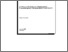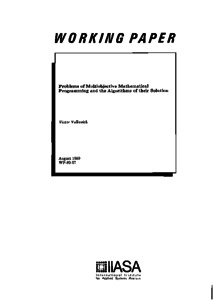Problems of Multiobjective Mathematical Programming and the Algorithms of their Solution

Volkovich, V. (1989). Problems of Multiobjective Mathematical Programming and the Algorithms of their Solution. IIASA Working Paper. IIASA, Laxenburg, Austria: WP-89-057Preview
Text
WP-89-057.pdf

Abstract

Development of interactive Decision Support Systems requires new approaches and numerical algorithms for solving Multiple Objective Optimization Problems. These algorithms must be robust and efficient and applicable to possibly a broad class of problems. This paper presents the new algorithm developed by the author. The algorithm consists of two steps: (a) reduction of the initial Multiple Objective Optimization Problem into a system of inequalities, and (b) solving this set of inequalities by the iterative procedure proposed by the author. Due to its generality, the algorithm applies to various Multiple Criteria Optimization Problems, including integer optimization problems. The author presents several variants of the algorithm as well as results of numerical experiments.

Item Type: Monograph (IIASA Working Paper) Methodology of Decision Analysis (MDA) IIASA Import 15 Jan 2016 01:59 27 Aug 2021 17:13 http://pure.iiasa.ac.at/3287View Item